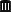ElMaestro
★★★

Denmark,
2021-08-23 16:42
(642 d 18:41 ago)

Posting: # 22532
Views: 3,030

## FDA guideline on BE, draft August 2021 [BE/BA News]

Hi all,

looks like FDA updated the canonical BE guideline for ANDAs. Draft.Pass or fail!
ElMaestro
Helmut
★★★Vienna, Austria,
2021-08-23 17:31
(642 d 17:52 ago)

@ ElMaestro
Posting: # 22533
Views: 2,431

## FDA guideline on BE, draft August 2021

Hi ElMaestro,

❝ Draft.What else…Main difference is that reference-scaling is detailed and not just mentioning the progesterone guidance in a footnote – like in thedraft of 2013. Interesting difference:

Progesterone guidance

$$\small{\text{a. the 95% upper confidence bound for }\left(\widehat{Y}_T-\widehat{Y}_R\right)^2-\theta s_{wR}^2\text{ must be}\leq 0}$$

New ANDA draft

$$\small{\text{a. The 95% upper confidence bound for }\left(\widehat{Y}_T-\widehat{Y}_R\right)^2-\theta s_{wR}^2\text{ must be}\leq 0}$$
$$\small{\;\;\;\;\text{(numbers should be kept to a minimum of four significant figures for comparison).}}$$

(my emphasis)
We had a few discussions about rounding in RSABE in the past (here and there).

In both guidances the formula is confusing. The regulatory limit $$\small{\sigma_\textrm{w0}=0.25}$$ leads to the regulatory constant $$\small{\theta_\textrm{s}=\frac{\log_{e}1.25}{\sigma_\textrm{w0}}=0.89257\ldots}$$Furthermore, we need the standard error $$\small{s_\textrm{d}}$$ of the point estimate $$\small{PE=\widehat{Y}_\textrm{T}-\widehat{Y}_\textrm{R}}$$ given by $$\small{s_\textrm{d}=\sqrt{\frac{MSE}{seq^2}\sum{\frac{1}{n_i}}},}$$ where $$\small{MSE}$$ is the residual Mean Squares Error, $$\small{seq}$$ the number of sequences, and $$\small{n_i}$$ are the number of subjects in sequence $$\small{i}$$. According to the SAS-code (without an explanation) $$\small{s_\textrm{d}}$$ acts as a bias correction and therefore,$$\small{crit=PE^2-s_\textrm{d}^2-\theta_\textrm{s}^2\cdot s_\textrm{wR}^2.}$$ Reference-scaling for NTIDs is also outlined. Again, a statement about rounding (contrary to the warfarin guidance).

Dif-tor heh smusma 🖖🏼 Довге життя Україна!Helmut SchützThe quality of responses received is directly proportional to the quality of the question asked. 🚮
Science Quotes
Achievwin
★

US,
2021-09-08 06:09
(627 d 05:14 ago)

@ Helmut
Posting: # 22560
Views: 1,890

## FDA guideline on BE, draft August 2021

❝ Furthermore, we need the standard error $$\small{s_\textrm{d}}$$ of the point estimate

I did not find this one in the guidance can you point out to me what line number this is in the new guidance?

Edit: quote restored [Ohlbe]
Helmut
★★★Vienna, Austria,
2021-09-10 22:47
(624 d 12:36 ago)

@ Achievwin
Posting: # 22567
Views: 1,785

## Bias correction unveiled

Hi Achievwin,

❝ ❝ Furthermore, we need the standard error $$\small{s_\textrm{d}}$$ of the point estimate

❝ I did not find this one in the guidance can you point out to me what line number this is in the new guidance?

That’s a little bit tricky. I’ll try again.

We have the regulatory constant $$\small{\theta_\textrm{s}=\frac{\log_{e}1.25}{\sigma_\textrm{w0}}=0.89257\ldots}\tag{1}$$
In the guidance line 998 the scaled average BE limit is given by $$\small{\theta\equiv\left(\frac{\log_{e}1.25}{\sigma_\textrm{w0}}\right)^2\tag{2}}$$which is simply what I used in my previous post by $$\small{\theta=\theta_\textrm{s}^2}\tag{3}$$ Of course, $$\small{\left(\widehat{Y}_T-\widehat{Y}_R\right)^2=PE^2}\tag{4}$$ In the guidance line 1003 we have $$\small{crit=\left(\widehat{Y}_T-\widehat{Y}_R\right)^2-\theta\cdot s_\textrm{wR}^2}\tag{5}$$ That’s the same as $$\small{crit=PE^2-\theta_\textrm{s}^2\cdot s_\textrm{wR}^2}\tag{6}$$Here the confusion starts. You find my $${\color{Red}{s_\textrm{d}}}$$ in lines 1083 and 1219 as the stderr of the difference.

In the respective next lines you find

x=estimate**2–stderr**2;

where it acts as a bias correction of the point estimate.

Hence, actually not $$(5)$$ or $$(6)$$ has to be used but $$\small{\begin{matrix} crit=PE^2-{\color{Red}{s_\textrm{d}^2}}-\theta_\textrm{s}^2\cdot s_\textrm{wR}^2 =\\ \left(\widehat{Y}_T-\widehat{Y}_R\right)^2-{\color{Red}{se^2}}-\theta \cdot s_\textrm{wR}^2 \end{matrix}}\tag{7}$$ The bias correction is not mentioned anywhere in the guidance and therefore, $$(5)$$ without one is extremely misleading.1,2 It is evident only if you inspect the SAS code. See Detlew’s post about the background given by Donald Schuirmann in 2016.

1. Troubles likely if one naïvely implements the formulas in another software…
2. This formula is used in all papers of Endrényi and Tóthfalusi.

Dif-tor heh smusma 🖖🏼 Довге життя Україна!Helmut SchützThe quality of responses received is directly proportional to the quality of the question asked. 🚮
Science Quotes
Achievwin
★

US,
2021-08-23 23:35
(642 d 11:48 ago)

@ ElMaestro
Posting: # 22534
Views: 2,297

## FDA guideline on BE, draft August 2021

❝ FDA updated the canonical BE guideline for ANDAs. Draft.Comments period is open till October 22, 2021, Therefore any issues bothering/benefit ANDA folks can be sent to FDA as comments for consideration.

FDA decided to split BA/BE guidance into two guidance 1) for ANDA filings and 2) for IND/NDAs

More elaborate explanations of each aspect.
Helmut
★★★Vienna, Austria,
2021-08-24 01:46
(642 d 09:37 ago)

@ Achievwin
Posting: # 22535
Views: 2,306

## FDA guideline on BE, draft August 2021

Hi Achievwin,

❝ FDA decided to split BA/BE guidance into two guidance 1) for ANDA filings and 2) for IND/NDAs

❝ More elaborate explanations of each aspect.

I expect to see an update of #2 (current draft of Feb 2019) as well.

Dif-tor heh smusma 🖖🏼 Довге життя Україна!Helmut SchützThe quality of responses received is directly proportional to the quality of the question asked. 🚮
Science QuotesIng. Helmut Schütz